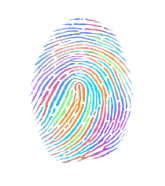Jens Segers on

# Perceptual image hashes

I recently discovered perceptual image hashes when I was in charge of removing thumbnails from a large set of images. Perceptual hashes are a completely different concept compared to the usual cryptographic hashing methods such as MD5 or SHA. With cryptographic hashes, a one-way digest is generated based on the input data. And because of its avalanche effect, the resulting hash is completely different when you change a single bit:

``````md5("10100110001") = 50144bd388adcd869bc921349a287690
md5("10100110101") = 3e3049b1ed21ede0237f9a276ec80703
``````

Because of this, the only way 2 images have the same cryptographic hash, is when they are exactly the same. This makes cryptographic hashing not a feasible solution to solve this problem.In contrast, a perceptual hash is a fingerprint based on the image input, that can be used to compare images by calculating the Hamming distance (which basically means counting the number of different individual bits).

There are a couple of different perceptual image hashing algorithms, but they all use similar steps for generating the media fingerprint. The easiest one to explain is the Average Hash (also called aHash). Let's take the following image and see how it works.### 1. Reduce size

First, we reduce the size of the image to 8x8 pixels. This is the fastest way to remove high frequencies and details. This step ignores the original size and aspect ratio, and will always resize to 8x8 so that we have 64 resulting pixels.### 2. Reduce the color

Now that we have 64 pixels, each with their RGB value, reduce the color by converting the image to grayscale. This will leave us with 64 color values.### 3. Calculate the average color

This is quite self-explanatory; calculate the average color using the previous 64 values.

### 4. Calculate the hash

The final fingerprint is calculated based on whether a pixel is brighter or darker than the average grayscale value we just calculated. Do this for every pixel and you end up with a 64 bit hash.

## Comparing images

To detect duplicate or similar images, calculate the perceptual hashes for both images:

``````Original:
1100100101101001001111000001100000001000000000000000011100111111
Thumbnail:
1100100101101001001111000001100000001000000000000000011100111111
``````

As you can see, both hashes are identical. But this doesn't mean that similar images will always create equal hashes! If we manipulate the original image, and add a watermark, we get these hashes:

``````Original:
1100100101101001001111000001100000001000000000000000011100111111
Watermark:
1100100101111001001111000001100000011000000010000000011100111111
``````

As you can see, these hashes are very similar, but not equal. To compare these hashes, we count the number of different bits (Hamming distance), which is 3 in this case. The higher this distance, the lower the change of identical or similar images.

If you have a MySQL database containing all hashes, you can do a lookup like this:

``````SELECT images.*, BIT_COUNT(hash ^ :hash) as hamming_distance
FROM images
HAVING hamming_distance < 5
``````

Note: because of the `BIT_COUNT` operation, InnoDB can not use an existing index on the hash column.

## Other implementations

The Average Hash implementation is the easiest and the fastest one, but it appears to be a bit too inaccurate and generates some false positives. Two other implementations are Difference Hash (or dHash) and pHash.

Difference Hash follows the same steps as the Average Hash, but generates the fingerprint based on whether the left pixel is brighter than the right one, instead of using a single average value. Compared to Average Hash it generates less false positives, which makes it a great default implementation.

pHash is an implementation that is quite different from the other ones, and does some really fancy stuff to increase the accuracy. It resizes to a 32x32 image, gets the Luma (brightness) value of each pixel and applies a discrete cosine transform (DCT) on the matrix. It then takes the top-left 8x8 pixels, which represent the lowest frequencies in the picture, to calculate the resulting hash by comparing each pixel to the median value. Because of it's complexity it is also the slowest one.

## PHP library

I combined and ported some implementations I found on the interwebs into a PHP package: https://github.com/jenssegers/imagehash

Feel free to contribute!